• OpenCV图像滤波重点摘抄图像滤波线性滤波非线性滤波 图像滤波 图像滤波 尽量保留图像细节特征的条件下对目标图像的噪声进行抑制 图像滤波的目的 一是抽出对象的特征作为图像识别的特征模式，二是为适应图像...
OpenCV图像滤波重点摘抄图像滤波线性滤波非线性滤波
图像滤波

图像滤波
尽量保留图像细节特征的条件下对目标图像的噪声进行抑制

图像滤波的目的
一是抽出对象的特征作为图像识别的特征模式，二是为适应图像处理的要求，消除图像数字化时所混入的噪声

图像滤波的要求
一是不能损坏图像的轮廓及边缘等重要信息，二是使图像清晰视觉效果好

滤波&模糊
滤波可分低通滤波和高通滤波两种：高斯滤波是指用高斯函数作为滤波函数的滤波操作，至于是不是模糊，要看是高斯低通还是高斯高通，低通就是模糊，高通就是锐化。

线性滤波非线性滤波展开全文opencv
• 本文实例讲述了Python图像滤波处理操作。分享给大家供大家参考，具体如下：在图像处理中，经常需要对图像进行平滑、锐化、边界增强等滤波处理。在使用PIL图像处理库时，我们通过Image类中的成员函数filter()来调用...
本文实例讲述了Python图像滤波处理操作。分享给大家供大家参考，具体如下：在图像处理中，经常需要对图像进行平滑、锐化、边界增强等滤波处理。在使用PIL图像处理库时，我们通过Image类中的成员函数filter()来调用滤波函数对图像进行滤波，而滤波函数则通过ImageFilter类来定义的。下面先直接看一个样例：#-*- coding: UTF-8 -*-from PIL import Imagefrom PIL import ImageFilterdef image_filters_test():im = Image.open("lena.jpg")#预定义的图像增强滤波器im_blur = im.filter(ImageFilter.BLUR)im_contour = im.filter(ImageFilter.CONTOUR)im_min = im.filter(ImageFilter.MinFilter(3))im.show()im_blur.show()im_contour.show()im_min.show()returnimage_filters_test()ImageFilter类中预定义了如下滤波方法：• BLUR：模糊滤波• CONTOUR：轮廓滤波• DETAIL：细节滤波• EDGE_ENHANCE：边界增强滤波• EDGE_ENHANCE_MORE：边界增强滤波(程度更深)• EMBOSS：浮雕滤波• FIND_EDGES：寻找边界滤波• SMOOTH：平滑滤波• SMOOTH_MORE：平滑滤波(程度更深)• SHARPEN：锐化滤波• GaussianBlur(radius=2)：高斯模糊>radius指定平滑半径。•UnsharpMask(radius=2, percent=150, threshold=3)：反锐化掩码滤波>radius指定模糊半径；>percent指定反锐化强度(百分比);>threshold控制被锐化的最小亮度变化。• Kernel(size, kernel, scale=None, offset=0)：核滤波当前版本只支持核大小为3×3和5×5的核大小，且图像格式为“L”和“RGB”的图像。>size指定核大小(width, height)；>kernel指定核权值的序列；>scale指定缩放因子；>offset指定偏移量，如果使用，则将该值加到缩放后的结果上。• RankFilter(size, rank)：排序滤波>size指定滤波核的大小；>rank指定选取排在第rank位的像素，若大小为0，则为最小值滤波；若大小为size * size / 2则为中值滤波；若大小为size * size – 1则为最大值滤波。• MedianFilter(size=3)：中值滤波>size指定核的大小• MinFilter(size=3)：最小值滤波器>size指定核的大小• MaxFilter(size=3)：最大值滤波器
展开全文• 一般的滤波器都是针对灰度图像的，scikit-image 库提供了针对彩色图像滤波的decorator：adapt_rgb，adapt_rgb 提供两种形式的滤波，一种是对rgb三个通道分别进行处理，另外一种方式是将rgb转为hsv颜色模型，然后针对...一般的滤波器都是针对灰度图像的，scikit-image 库提供了针对彩色图像滤波的decorator：adapt_rgb，adapt_rgb 提供两种形式的滤波，一种是对rgb三个通道分别进行处理，另外一种方式是将rgb转为hsv颜色模型，然后针对v通道进行处理，最后再转回rgb颜色模型。针对模式一，称为 each_channel@adapt_rgb(each_channel)def sobel_each(image):return filters.sobel(image)模式二称为 hsv_value@adapt_rgb(hsv_value)def sobel_hsv(image):return filters.sobel(image)利用上述两种模式，可以对彩色图像滤波，下面是完整的用例代码;from skimage import datafrom skimage.exposure import rescale_intensityimport matplotlib.pyplot as pltfrom skimage.color.adapt_rgb import adapt_rgb, each_channel, hsv_valuefrom skimage import filters@adapt_rgb(each_channel)def sobel_each(image):return filters.sobel(image)@adapt_rgb(hsv_value)def sobel_hsv(image):return filters.sobel(image)image = data.astronaut()# display the original imageplt.imshow(image)fig = plt.figure(figsize=(16, 9))ax_each = fig.add_subplot(121, adjustable='box-forced')ax_hsv = fig.add_subplot(122, sharex=ax_each, sharey=ax_each, adjustable='box-forced')# We use 1 - sobel_each(image)# but this will not work if image is not normalizedax_each.imshow(rescale_intensity(1 - sobel_each(image)))ax_each.set_xticks([]), ax_each.set_yticks([])ax_each.set_title("Sobel filter computed\n on individual RGB channels")# We use 1 - sobel_hsv(image) but this will not work if image is not normalizedax_hsv.imshow(rescale_intensity(1 - sobel_hsv(image)))ax_hsv.set_xticks([]), ax_hsv.set_yticks([])ax_hsv.set_title("Sobel filter computed\n on Value converted image (HSV)")plt.show()参考来源：原图：效果图:(责任编辑：ioter)
展开全文• 文章目录图像滤波与增强一、图像滤波简介 图像滤波与增强 一、图像滤波简介 1、
文章目录图像滤波与增强一、图像滤波简介二、线性滤波2.1 方框滤波2.2 均值滤波2.3 高斯滤波三、非线性滤波3.1 中值滤波3.2 双边滤波四、图像增强4.1 图像锐化4.2 直方图均衡化4.3 Gamma变化
图像滤波与增强
一、图像滤波简介
1、滤波实际上是信号处理的一个概念，图像可以看成是一个二维信号，其中像素点的灰度值代表信号的强弱；
2、高频：图像上变化剧烈的部分；低频：图像灰度值变换缓慢，平坦的地方；
3、根据图像高低频，设置高通和低通滤波器。高通滤波器可以检测变化尖锐，明显的地方，低通可以让图像变得平滑，消除噪声；
4、滤波作用：高通滤波用于边缘检测，低通滤波用于图像平滑去噪；
5、滤波包括线性滤波(方框滤波/均值滤波/高斯滤波)和非线性滤波(中值滤波/双边滤波)。
二、线性滤波
说到线性滤波，首先得先提一下邻域算子，邻域算子就是利用给定像素周围的像素值决定此像素的最终输出值的一种算子。线性滤波是一种常用的邻域算子，像素输出取决于输入像素的加权和：
$g(i, j) = \Sigma_{k, l}f(i+k, j+l)h(k, l)$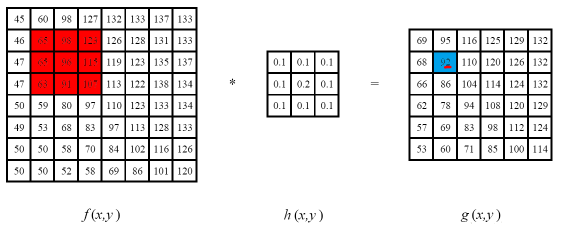2.1 方框滤波
方框滤波(box Filter)被封装在一个名为boxFilter的函数中，即boxFilter函数的作用是使用方框滤波器(box filter)来模糊一张图片，从src输入，从dst输出；
方框滤波核：
$K = \alpha \left[ \begin{matrix} 1 & 1 & 1 &...& 1 &1 \\ 1 & 1 & 1 & ... & 1 & 1 \\ ...&...&...&...&...&... \\ 1 & 1 & 1 & ... & 1 & 1 \end{matrix} \right]$
$\alpha = \begin{cases} \frac{1}{width*height} \quad normalize=true \\ \quad \quad \ 1 \quad \quad \quad normalize=false \end{cases}$

方框滤波函数：
cv2.boxFilter(src, depth, ksize, normalize)
参数说明：
参数1：输入图像
参数2：目标图像深度
参数3：核大小
参数4：normalize属性

import cv2
import numpy as np

img = cv2.imread('images/lena.png')
r = cv2.boxFilter(img, -1, (7, 7), normalize=1)
d = cv2.boxFilter(img, -1, (3, 3), normalize=0)
cv2.namedWindow('img', cv2.WINDOW_AUTOSIZE)
cv2.namedWindow('r', cv2.WINDOW_AUTOSIZE)
cv2.namedWindow('d', cv2.WINDOW_AUTOSIZE)
cv2.imshow('img', img)
cv2.imshow('r', r)
cv2.imshow('d', d)
cv2.waitKey(0)
cv2.destroyAllWindows()

2.2 均值滤波
均值滤波是一种最简单的滤波处理，它取的是卷积核区域内元素的均值，用cv2.blur()实现，如3*3的卷积核：
$kernel=\frac{1}{9} \left[ \begin{matrix} 1 & 1 & 1\\ 1 & 1 & 1 \\ 1 & 1 & 1 \end{matrix} \right]$

均值滤波函数：
cv2.blur(src, ksize)
参数说明：
参数1：输入图像
参数2：kernel的大小，一般为奇数

import cv2
import numpy as np
from matplotlib import pyplot as plt

img = cv2.imread('images/opencv.png')
cv2.imshow('img', img)
cv2.waitKey(0)
cv2.destroyAllWindows()
img = cv2.cvtColor(img, cv2.COLOR_BGR2RGB)
blur = cv2.blur(img, (3, 3))
plt.subplot(121), plt.imshow(img), plt.title('Original')
plt.xticks([]), plt.yticks([])
plt.subplot(122), plt.imshow(blur), plt.title('Blurred')
plt.xticks([]), plt.yticks([])
plt.show()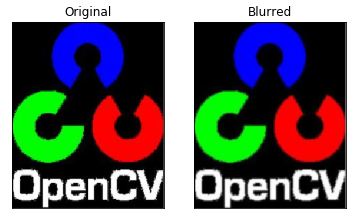2.3 高斯滤波
高斯滤波是一种线性平滑滤波，适用于消除高斯噪声，广泛应用于图像处理的减噪过程。高斯滤波的卷积核权重并不相同，中间像素权重最高，越远离中心的像素权重越小。其原理是一个2维高斯函数。高斯滤波相比均值滤波效率要慢，但可以有效消除高斯噪声，能保留更多的图像细节，所以被经常称为最有用的滤波器。

高斯滤波函数：
cv2.Guassianblur(src, ksize, std)
参数说明：
参数1：输入原图
参数2：高斯核大小
参数3：标准差σ，平滑时，调整σ实际是在调整周围像素对当前像素的影响程度，调大σ即提高了远处像素对中心像素的影响程度，滤波结果也就越平滑。

import cv2
import numpy as np
from matplotlib import pyplot as plt

img = cv2.imread('images/median.png')
img = cv2.cvtColor(img, cv2.COLOR_BGR2RGB)
blur = cv2.GaussianBlur(img, (7, 7), 7)
plt.subplot(121), plt.imshow(img), plt.title('Original')
plt.xticks([]), plt.yticks([])
plt.subplot(122), plt.imshow(blur), plt.title('Blurred')
plt.xticks([]), plt.yticks([])
plt.show()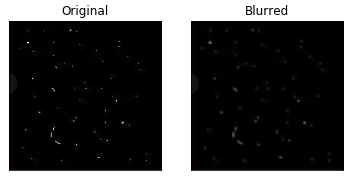三、非线性滤波
3.1 中值滤波
中值滤波是一种非线性滤波，使用像素点邻域灰度值的中值指代该点的灰度值，中值滤波可以去除椒盐噪声和斑点噪声。

中值滤波函数：
cv2.medianBlur(img, ksize)
参数说明：
参数1：输入原图
参数2：核大小

import cv2
import numpy as np
import matplotlib.pyplot as plt

img = cv2.imread('images/median.png')
median = cv2.medianBlur(img, 3)
plt.subplot(121), plt.imshow(img), plt.title('Original')
plt.xticks([]), plt.yticks([])
plt.subplot(122), plt.imshow(median), plt.title('median')
plt.xticks([]), plt.yticks([])
plt.show()

3.2 双边滤波
双边滤波是一种非线性滤波的方法，是结合图像的空间邻近度和像素值相似度的一种折中处理，同时考虑空间与信息和灰度相似性，达到保边去噪的目的，具有简单、非迭代、局部处理的特点。

双边滤波函数：
cv2.bilateralFilter(src=image, d, sigmaColor, sigmaSpace)
参数说明：
参数1：输入图像
参数2：像素的邻域直径
参数3：灰度值相似性高斯函数标准差
参数4：空间高斯函数标准差

关于两个sigma参数，简单起见，可以另两个sigma的值相等；如果它们很小(小于10)，那么滤波器几乎没什么效果；如果它们很大(大于150)，那么滤波器效果就会很强，使图像非常卡通化；
关于参数d，过大(d>5)执行效率低。对于实时应用，建议取d=5；对于需要过滤严重噪声的离线应用，可取d=9；d>0时，由d指定邻域直径；d<=0时，d会自动由sigmaSpace的值确定，且d与sigmaSpace成正比。
import cv2
from matplotlib import pyplot as plt

img = cv2.imread('images/bilateral.png')
img = cv2.cvtColor(img, cv2.COLOR_BGR2RGB)
blur = cv2.bilateralFilter(img, -1, 150, 100)
plt.subplot(121), plt.imshow(img), plt.title('Original')
plt.xticks([]), plt.yticks([])
plt.subplot(122), plt.imshow(blur), plt.title('Blurred')
plt.xticks([]), plt.yticks([])
plt.show()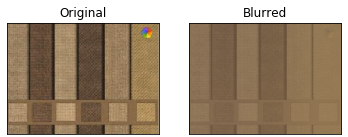四、图像增强
4.1 图像锐化
图像锐化与图像平滑时相反的操作，锐化是通过增强高频分量来较少图像中的模糊，增强图像细节边缘和轮廓，增强灰度反差，便于后期对目标的识别和处理。锐化处理在增强图像边缘的同时也增加了图像的噪声。方法通常用微分法和高通滤波法。
import cv2
import numpy as np

def custom_blur_demo(image):
kernel = np.array([[0, -1, 0], [-1, 5, -1], [0, -1, 0]], np.float32) # 锐化
dst = cv2.filter2D(image, -1, kernel=kernel)
cv2.imshow('custom_blur_demo', dst)

src = cv2.imread('images/sharpen.png')
# cv2.namedWindow('input image', cv2.WINDOW_AUTOSIZE)
cv2.imshow('input image', src)
custom_blur_demo(src)
cv2.waitKey(0)
cv2.destroyAllWindows()4.2 直方图均衡化
直方图均衡化是将原图像通过某种变换，得到一幅灰度直方图为均匀分布的新图像的方法。
直方图均衡化方法的基本思想是对在图像中像素个数多的灰度级进行展宽，而对像素少的灰度级进行缩减。从而达到清晰图像的目的。

直方图均衡化函数：
cv2.equalizeHist(img)
参数：待均衡化图像

步骤：
a.统计直方图中每个灰度级出现的次数；
b.计算累计归一化直方图；
c.重新计算像素点的像素值。

import cv2
import matplotlib.pyplot as plt

# 直接读取灰度图像
img = cv2.imread('./images/dark.png', 0)
cv2.imshow('dark', img)
# 调用cv2.equalizeHist函数进行直方图均衡化
img_equal = cv2.equalizeHist(img)
cv2.imshow('img_equal', img_equal)
cv2.waitKey(0)
cv2.destroyAllWindows()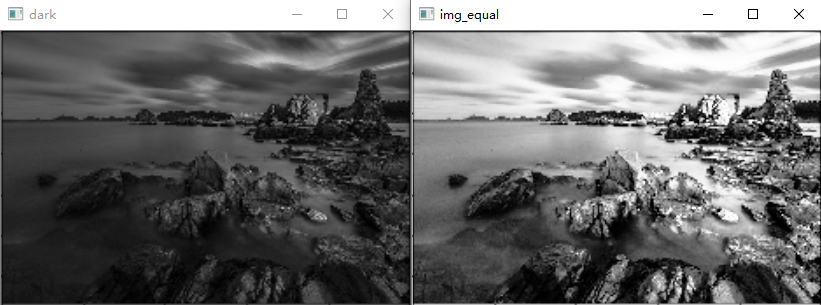import cv2
import numpy as np

img = cv2.imread('./images/dark1.jpg')
cv2.imshow('src', img)
# 彩色图像均衡化，需要分解通道，对每一个通道均衡化
(b, g, r) = cv2.split(img)
bH = cv2.equalizeHist(b)
gH = cv2.equalizeHist(g)
rH = cv2.equalizeHist(r)
# 合并每一个通道
result = cv2.merge((bH, gH, rH))
cv2.imshow('dst', result)
cv2.waitKey(0)
cv2.destroyAllWindows()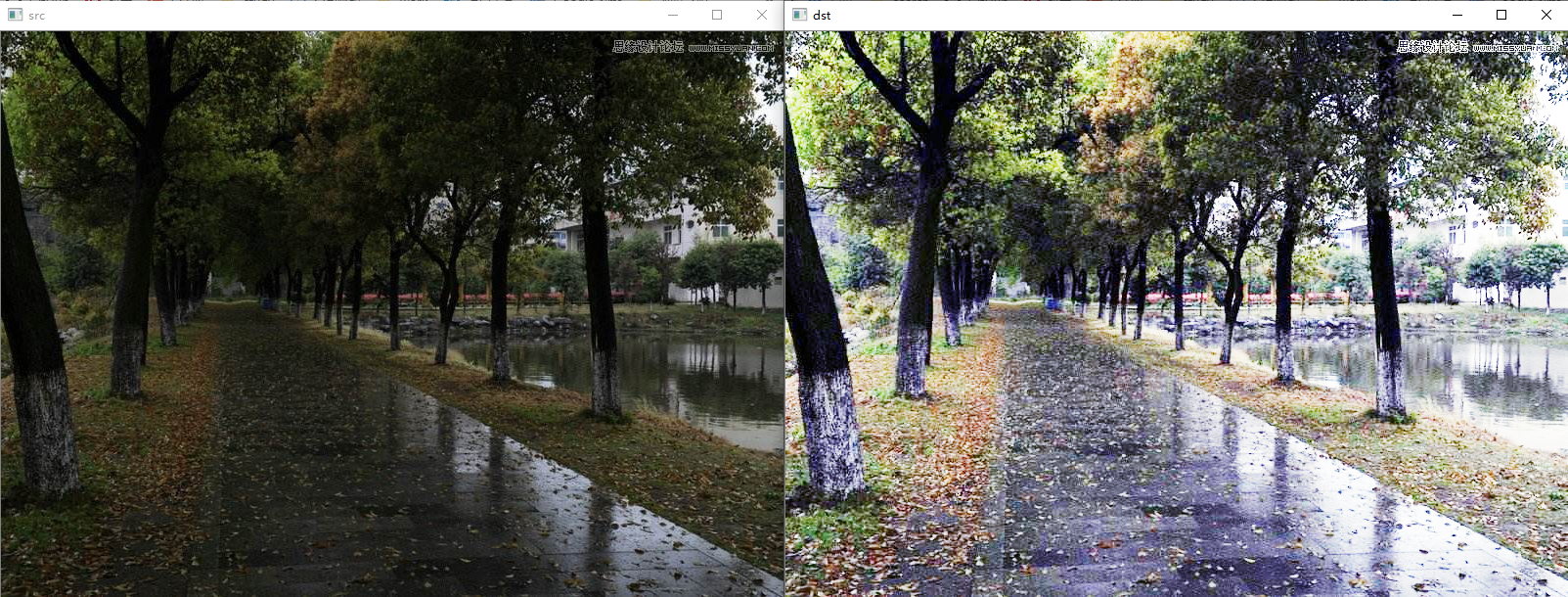自己动手实现一下直方图均衡化算法：
import numpy as np
import cv2

def hist_equalization(matrix):
dict_pix = {}
dict_dst = {}
dst = np.zeros_like(matrix)
for i in range(len(matrix)):
for j in range(len(matrix)):
dict_pix[matrix[i, j]] = dict_pix.get(matrix[i, j], 0) + 1
pixs = sorted(dict_pix.keys())
sumPi = 0
for pix in pixs:
image = matrix.shape * matrix.shape
Pi = dict_pix[pix]/image
sumPi += Pi
result = sumPi * 256 - 1 if sumPi * 256 -1 > 0 else 0
dict_dst[pix] = round(result)
for i in range(len(matrix)):
for j in range(len(matrix)):
dst[i, j] = dict_dst[matrix[i, j]]
return dst

4.3 Gamma变化
Gamma变换是对输入图像灰度值进行的非线性操作，是输出图像灰度值与输入图像灰度值成指数关系：
$V_{out} = AV_{in}^\gamma$
Gamma变换就是用来图像增强，其提升了暗部细节，通过非线性变换，让图像从曝光强度的线性响应变得更接近人眼感受的响应，即将漂白(相机曝光)或过暗(曝光不足)的图片，进行矫正。
import cv2
import numpy as np

img = cv2.imread('./images/dark1.jpg')

def adjust_gamma(image, gamma=1.0):
invGamma = 1.0 / gamma
table = []
for i in range(256):
table.append(((i / 255.0) ** invGamma) * 255)
table = np.array(table).astype('uint8')
#     print(table)
return cv2.LUT(image, table)

img_gamma = adjust_gamma(img, 1.5)
# print(img_gamma)
cv2.imshow('img', img)
cv2.imshow('img_gamma', img_gamma)

cv2.waitKey(0)
cv2.destroyAllWindows()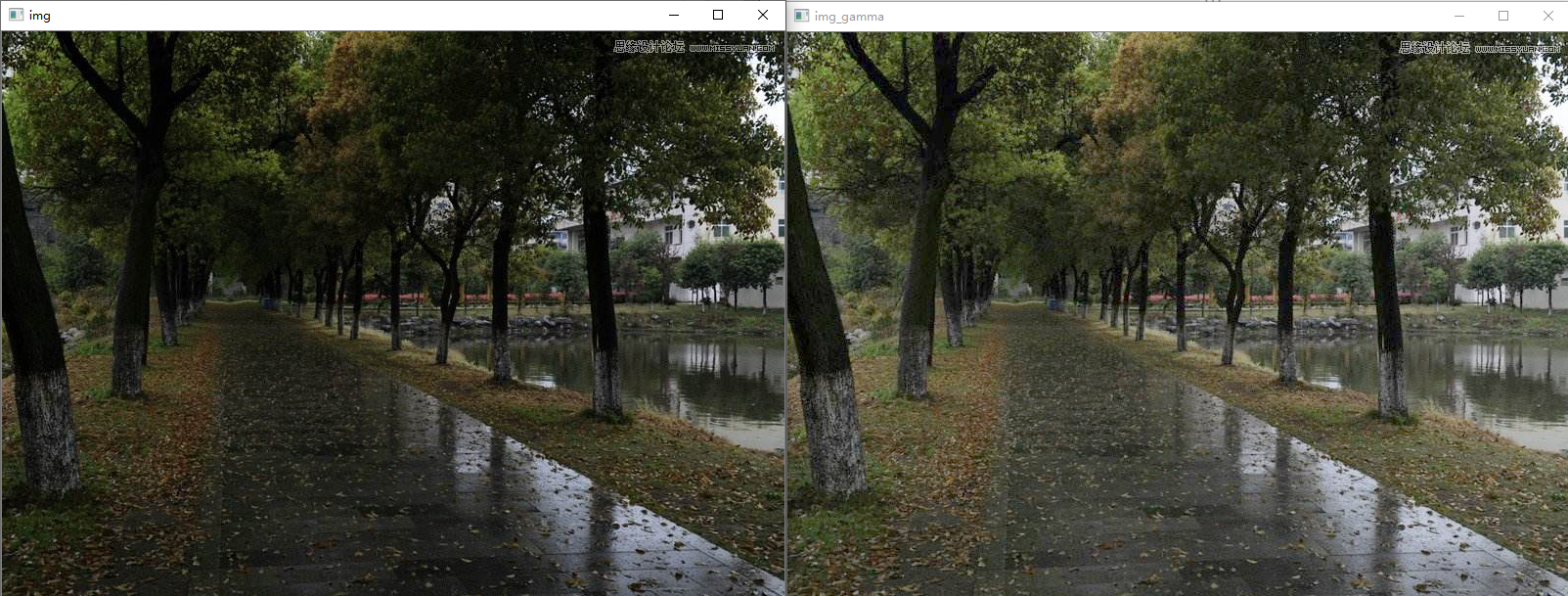展开全文• ## 图像滤波算法总结

万次阅读 多人点赞 2018-09-11 00:01:13
该篇主要是对图像滤波算法一个整理，主要参考的大神的博客： https://blog.csdn.net/qq_15606489/article/details/52755444 1：图像滤波既可以在实域进行，也可以在频域进行。图像滤波可以更改或者增强图像。通过...
• 图像滤波图像滤波图像滤波 作用:去除图像中的干扰（噪声)。噪声一般是由外界干扰产生的，比如灰尘、热噪声等。均值滤波:对于模糊图像以及图像内部的噪声具有很好的作用。 滤波模板是描述滤波器的大小，里边存储着...
• 1、图像滤波的分类和基本概念 概念 图像滤波，即在尽量保留图像细节特征的条件下对目标图像的噪声进行抑制，是图像预处理中不可缺少的操作。 图像滤波的目的有两个： 1、抽出对象的特征作为图像识别的特征模式； 2、...计算机视觉
• 图像滤波原理之双边滤波图像滤波问题描述1. 空间(即图像定义域)相邻关系的滤波器2. 双边滤波器（bilateral filter）3. 如何快速实现双边滤波 图像滤波问题描述 为了内容的完整性，首先对图像滤波进行简单的回顾。...
• 图像滤波与卷积 与1维信号滤波类似，图像滤波由卷积定义 在图像中，也常以模版的形式定义 如果滤波器对称，则二者等价 某一像素滤波结果 计算过程 图像去噪（图像平滑） 图像平滑—平均滤波 在...
• 图像处理，二维图像滤波之维纳滤波源代码，课程实验Matlab
• 图像滤波，即在尽量保留图像细节特征的条件下对目标图像的噪声进行抑制，是图像预处理中不可缺少的操作，其处理效果的好坏将直接影响到后续图像处理和分析的有效性和可靠性。 图像滤波的主要目的：为了消除图像中...
• 针对目标检测跟踪的图像滤波算法的评价,提出了目标与背景的交叉分辨力评价算子及评价准则。仿真实验表明,该滤波算法要优于中值滤波、自适应滤波、小波变换滤波算法,滤波质量的定量评价算法是合理的、有效的。算法...
• MATLAB图像滤波去噪分析及其应用.MATLAB图像滤波去噪分析及其应用MATLAB
• %实现空间滤波领域处理时的一个重要考虑因素，当滤波掩模的中心靠近图像轮廓时发生的情况 % 当一个n*n的方形掩模，其中心距离图像边缘为(n-1)/2个像素时，该掩模至少有一条边与图像轮廓相重合 %当掩模的中心继续向......